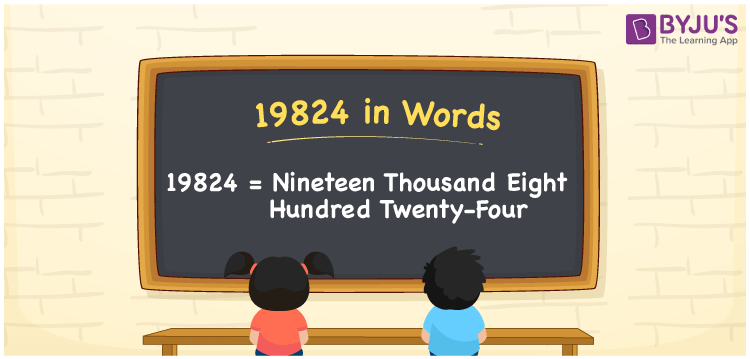# 19824 in Words

19824 in words can be written as Nineteen Thousand Eight Hundred Twenty-four. The article of 19824 in words will provide students with a clear knowledge of the concept of counting numbers. If Rs. 19824 is spent on buying a new bicycle, then you can say that “I spent Nineteen Thousand Eight Hundred Twenty-four Rupees in buying a new bicycle”. The  concept of converting 19824 into words using the English alphabet is discussed here. Students will be able to convert numbers in words with the help of the resources available at BYJU’S. 19824 can be read as “Nineteen Thousand Eight Hundred Twenty-four” in English.

 19824 in words Nineteen Thousand Eight Hundred Twenty-four Nineteen Thousand Eight Hundred Twenty-four in Numbers 19824

## 19824 in English Words## How to Write 19824 in Words?

In this section you will learn in detail about conversion of 19824 into words using the place value chart. Five digits are present in 19824. The place value chart of 19824 can be understood easily using the table below.

 Ten Thousands Thousands Hundreds Tens Ones 1 9 8 2 4

The expanded form of the number 19824 is given below:

1 x Ten Thousand + 9 × Thousand + 8 × Hundred + 2 × Ten + 4 × One

= 1 x 10000 + 9 × 1000  + 8 × 100 + 2 × 10 + 0 × 4

= 10000 + 9000 + 800 + 20 + 4

= 19824

= Nineteen Thousand Eight Hundred Twenty-four

Hence, 19824 in words is written as Nineteen Thousand Eight Hundred Twenty-four.

19824 is a natural number that precedes 19825 and succeeds 19823.

19824 in words – Nineteen Thousand Eight Hundred Twenty-four

Is 19824 an odd number? – No

Is 19824 an even number? – Yes

Is 19824 a perfect square number? – No

Is 19824 a perfect cube number? – No

Is 19824 a prime number? – No

Is 19824 a composite number? – Yes

## Frequently Asked Questions on 19824 in Words

Q1

### Write 19824 in words.

19824 can be written as “Nineteen Thousand Eight Hundred Twenty-four” in words.
Q2

### Is 19824 an odd or even number?

19824 is an even number because it is divisible by 2.
19824/2 = 9912
Q3

### Write Nineteen Thousand Eight Hundred Twenty-four in numbers.

Nineteen Thousand Eight Hundred Twenty-four can be written in numbers as 19824.